UPSC  >  Character Puzzles Questions with Answers

# Character Puzzles Questions with Answers - Notes | Study CSAT Preparation for UPSC CSE - UPSC

 1 Crore+ students have signed up on EduRev. Have you?

Basics for solution:
In this type of questions. a figure or a matrix is given in which some numbers are filled according to a rule. A place is left blank. You have to find out a character (a number or a letter) from the given possible answers which may be filled in the blank space.
Some examples are Riven below:
Example 1:
Which number will replace the question mark?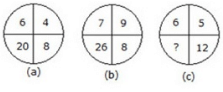Solution:
From fig. a: 6 + 4 + 8 = 18
18 + 2 = 20
From flg. b: 7 + 9 + 8 = 24
24 + 2 = 26
From fig. c: 6 + 5 + 12 = 23
23+2 = 25
Hence the number 25 will replace the question mark.

Example 2:
Which number will replace the question mark?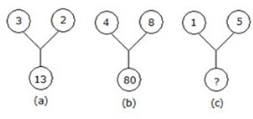Solution:
From flg. a: (3)2 + (2)2 = 13
From fig. b: (4)+ (8), = 80
From fig. c: ? = (1)2 + (5)2
? = 1 + 25
? = 26
Hence the number 26 will replace the question mark.

Example 3:
Which number will replace the question mark?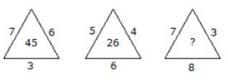Solution:
From fig. a: 7 x 6 + 3 = 45
From fig. b: 5 x 4 + 6 = 26
From fig. c: 7 x 3 + 8 = 29
Hence the number 29 will replace the question mark.

Example 4:
Which number will replace the question mark?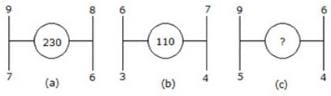Solution:
From fig. a: 92 + 82 + 72 + 62 = 81 +64 + 49 + 36 = 230
From fig. b: 6+ T+ 3+ 42 = 36 + 49 + 9 + 16 = 110
From fig. c: 92+ 6+ 52 + 4= 81 + 36 + 25 + 16= 158
Hence the number 158 will replace the question mark.

Example 5:
Which number will replace the question mark?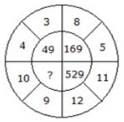Solution:
(4+3)=(7)=49
(8 + 5)2 = (13)= 169
(11 + 12)2 = (23)2 = 529
(10 + 9)2 = (19)2 = 361
Hence the number 361 will replace the questich mark.

Example 6:
Which number will replace the question mark?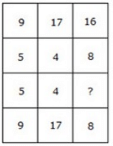Solution:
From column is I: (9 x 5) % 5 = 9
From column II: (17 x 4) % 4 = 17
From column III: (16 x ?) % 8 = 8
16? = 64
? = 4
Hence the number 4 will replace the question mark.

Example 7:
Which number will replace the question mark?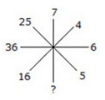Solution:
(5)2 = 25
(6)2 = 36
(4)= 16
(7)2 = 49
Hence the number 49 will replace the question mark.

The document Character Puzzles Questions with Answers - Notes | Study CSAT Preparation for UPSC CSE - UPSC is a part of the UPSC Course CSAT Preparation for UPSC CSE.
All you need of UPSC at this link: UPSC

## CSAT Preparation for UPSC CSE

72 videos|64 docs|92 tests

## CSAT Preparation for UPSC CSE

72 videos|64 docs|92 tests

### How to Prepare for UPSC

Read our guide to prepare for UPSC which is created by Toppers & the best Teachers

Track your progress, build streaks, highlight & save important lessons and more!

,

,

,

,

,

,

,

,

,

,

,

,

,

,

,

,

,

,

,

,

,

;